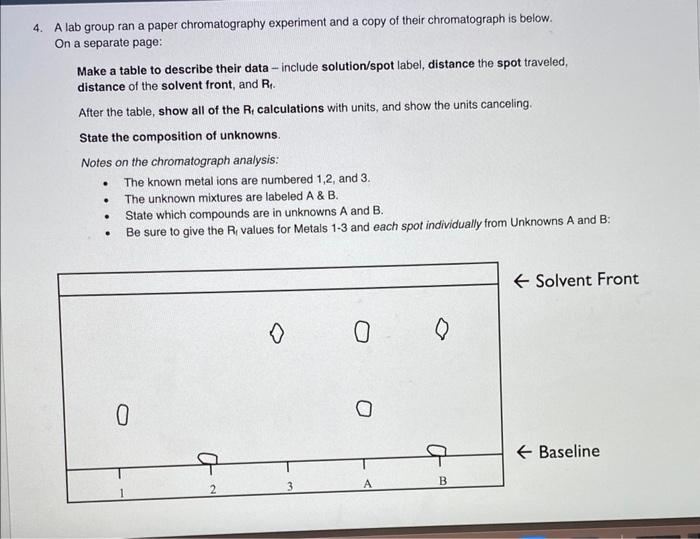Home / Expert Answers / Chemistry / help-on-the-table-and-calculations-pls-a-lab-group-ran-a-paper-chromatography-experiment-and-a-copy-pa170

# (Solved): help on the table and calculations pls A lab group ran a paper chromatography experiment and a copy ...

help on the table and calculations plsA lab group ran a paper chromatography experiment and a copy of their chromatograph is below. On a separate page: Make a table to describe their data - include solution/spot label, distance the spot traveled, distance of the solvent front, and $$\mathrm{R}_{\mathrm{t}}$$. After the table, show all of the $$\mathrm{R}_{\mathrm{t}}$$ calculations with units, and show the units canceling. State the composition of unknowns. Notes on the chromatograph analysis: - The known metal ions are numbered 1,2, and $$3 .$$ - The unknown mixtures are labeled A \& B. - State which compounds are in unknowns $$A$$ and $$B$$. - Be sure to give the $$R_{i}$$ values for Metals 1-3 and each spot individually from Unknowns A and B:

We have an Answer from Expert